Search IntMath
Close

450+ Math Lessons written by Math Professors and Teachers

5 Million+ Students Helped Each Year

1200+ Articles Written by Math Educators and Enthusiasts

Simplifying and Teaching Math for Over 23 Years

# IntMath Newsletter: 3-D conics, interactives, March math

By Murray Bourne, 12 Mar 2012

12 Mar 2012

1. Interactive 3-D conic sections graph
2. List of math interactives on IntMath.com
3. Special math events in March
4. Math Puzzles
5. Recent popular tweets
6. Friday math movies
7. Final thought: the greatest mistake

## 1. Interactive 3-D conic sections graph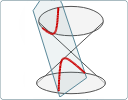Here's a new 3-D interactive graph. You can use it to see how we get circles, ellipses, parabolas and hyperbolas from intersecting a double cone by a plane. Interactive 3-D conic sections graph

## 2. List of math interactives on IntMath.com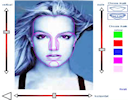I recently compiled a list of all the math manipulatives on IntMath.com. These interactives are designed to help users understand math concepts through exploring. Here they all are: List of math interactives on IntMath.com

## 3. Special math events in March

a. Pi Day (14 Mar)

The number pi = 3.141592... arises in many different fields of mathematics, not just in circles!

Mar 14 (3.14) is celebrated as Pi Day. Meanwhile, some mathematicians think we should drop pi.

Researchers Yee and Kondo claim to have calculated Pi to 10 trillion digits on 17 Oct 2011.

b. Brain Awareness Week (12 to 18 Mar, 2012)

Brain Awareness Week aims to promote the public and personal benefits of brain research. It's organized by the Dana Alliance for Brain Initiatives and Society for Neuroscience

c. Expanding Your Horizons

The mission of Expanding Your Horizons Network is to encourage young women to pursue science, technology, engineering and mathematics (STEM) careers. This organization is quite active around International Women's Day (8th Mar).

You may have noticed Google used the following image on its home page on that day: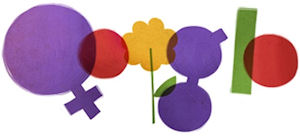d. Birthdays

Here are some mathematically significant March birthdays:

1. Jules Antoine Lissajous, French mathematician, famous for the interesting curves called Lissajous Figures (born 4 Mar 1822)
2. Gerardus Mercator, map maker, known for the Mercator Projection world map (born 5 March 1512)
3. Albert Einstein (born 14 Mar 1879)
4. René Descartes, mathematician and philosopher, developer of the cartesian x-y coordinate system (born 31 Mar 1596)

## 4. Math Puzzles

There were plenty of responses to the puzzle I presented in the last IntMath Newsletter about Egyptian fractions.

The correct answer, 1/2 + 1/3 + 1/78, was given by Don, Tomas, Laureli, Greg, Arun, George and Saran.

Special mention goes to to Francis and Sivy who were correct, but also showed the thinking behind their answers.

New puzzle: A man asked a zookeeper how many beasts and how many birds there were in his zoo. The zookeeper (a frustrated mathematician), answered, "There are 30 heads and 100 feet."

How many animals and birds were there? Please respond here.

## 5. Recent popular tweets

These tweets got a lot of response on Twitter:

1. Here's a list of free math lectures: 100 Incredible Open Lectures for Math Geeks (the link has since gone down!)
2. This one is good for Pi Day:
A pizza with radius "z" and height "a" has volume pi*z*z*a.

## 6. Friday math movies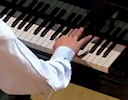(a) The world's ugliest music All music has a mathematical framework, but some music starts with a math concept. Here's an example. Friday math movie: The world's ugliest music(b) How to make choosing easier Sheena Iyengar gives plenty of examples in this talk where we learn when it comes to choices, less is more. This video has many implications for more effective teaching. Friday math movie: How to make choosing easier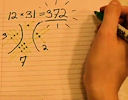(c) Vi Hart Visual Multiplication and 48/2(9+3) This short video explores an alternate way to do multiplication. Friday math movie: Vi Hart Visual Multiplication and 48/2(9+3)

## 7. Final thought: the greatest mistake

Mathematics, like most activities involving problem solving, requires us to get into it and get our hands dirty - and of course, learn by making mistakes.

The following gem comes from American writer, publisher, artist, and philosopher Elbert Hubbard.

The greatest mistake you can make in life is to be continually fearing you will make one. [Elbert Hubbard]

Until next time, enjoy whatever you learn.

See the 26 Comments below.

### 26 Comments on “IntMath Newsletter: 3-D conics, interactives, March math”

1. Christopher Buchanan says:

a + b = 30
4a + 2b = 100
solve simultaneously: a = 20, b = 10

2. Bozo says:

A man asked a zookeeper how many beasts and how many birds there were in his zoo. The zookeeper (a frustrated mathematician), answered, "There are 30 heads and 100 feet."
Sol: let x= no of birds and y = no of animals
2x+4y=100
x+y=30
solution set is {10,20}

3. Bill Williamson says:

The interactive graph on conic sections was absolutely superb.
Well done

4. Avinash Dhinwa says:

Number of beasts = 20
Number of birds = 10

5. Muhammad Anwar Khan says:

i have solved the puzzle by using two simultaneous equations

4X+2Y=100 2X+y=50
and
X+Y=30
X=20(animals)
Y=10 (Birds)

6. Guido Verbraeken says:

Birds are animals too.
Let X : number of animals with 4 feet
let Y : number of animals with 2 feet

then : X + Y = 30 |-2| -2X - 2Y = -60
4X +2Y = 100 | 1| 4X + 2Y = 100
---------------
2X = 40
X = 20
and Y = 10
So there are 30 animals all together of which 10 birds

7. Alex Green says:

Let a be the number of animals
Leb b be the number of birds

a + b = 30 ---(1) {since there are 30 heads}
4a + 2b = 100---(2)
{since there are 100 feet, assuming that all beasts have 4 legs and all birds have 2 legs}

Multiplying eqn(1) by 2 and subtracting from eqn(2) we get:
2a = 40, hence a = 20
Sub a = 20 into eqn(1) we get 20 + b = 30, hence b = 10

Conclusion: There are 20 animals and 10 birds

8. Zeeshan says:

20 beasts
10 birds
Solution:
let x beasts and y birds
so
x+y==30
4x+2y==100
by solving simultaneously we get
x==20,y==10

9. Dishant says:

The Answer to the Puzzle is :- 20 Beasts and 10 Birds..:)

10. Laureli Sluggett says:

x=beasts
y=birds
x+y=30
4x+2y=100
== 20 beasts 10 birds (20x4)+(10x2)=100 & 20+10=30

: )

11. Devin says:

There are 20 beasts and 10 birds in the zoo.

**
Assuming all animals have 1 head, each beast has 4 feet and birda have 2.
X = # of beasts, Y = # of birds
X + Y = 30
X = 30 - Y

4X + 2Y = 100
2X + Y = 50
2(30 - Y) + Y = 50
Y = 10
X = 20

12. Wynill says:

60 animals and 30 birds

13. gfrblxt says:

30 heads + 100 feet --> 20 beasts (4-legged), 10 birds (2-legged). Classic Diophantine equation problem - love it! Assuming that the zoo had no pushme-pullyus 🙂

14. Steven Nckerson says:

Ans to puzzle is 20 animals and 10 birds. No real math required as each animal has at least 2 legs, so giving each entity 2 legs uses 60 legs with 40 legs remaining. Thats enough to go back and give 20 entities 2 more legs, so 20 get 4 and 10 get only 2.

15. Tomas Garza says:

Assuming snakes, snails, fish, and all other footless beasts are excluded, then if x stands for number of birds and y for number of beasts, one has to solve the following two equations: Number of heads (number of birds plus number of beasts) equals 30, and Number of feet (2 for each bird and 4 for each beast) equals 100. I.e., x + y = 30; 2x + 4y = 100. Then number of birds is 10 and number of beasts is 20.

16. inday aguada says:

17. Shau says:

beasts;x
birds; 30-x

4x + 2(30-x)=100
x+30= 50
x=20

Beasts=20
Birds=10

18. Wilna Myburgh says:

I am a Mathis teacher and love your site. It is interesting and gives me lots of inspiration to teach a subject that is often perceived by struggling students as boring and hard. Thanks for a great website.

19. Murray says:

@Bill: Thanks - glad you liked the conic sections interactive graph. (I only made some modifications to Lodewijk Bogaards' great script.)

20. Murray says:

@Wilna: Thanks for your kind comments. Glad you find IntMath useful!

21. anthonette says:

let x=beast, y=birds
since there are 30 heads and assuming all of them have only one head,
(1) x+y=30 => y=30-x (5)
and since beasts have 4 legs and birds have 2 legs,
(2)4x+2y=100 => 2x+y=50 (4)

substituting equation (5) to equation (4):
2x+y=50
2x+(30-x)=50
x=50-30
x=20

if x=20;
y=30-x
y=30-20
y=10

therefore, there are 20 beasts and 10 birds in the zoo.. 🙂

22. Laaj says:

simmply 20 beasts and 10 birds..:)

23. Ricky says:

Let no. of beasts=a
& no. of birds=b
if we assume beasts to be 4-legged & birds to be 2-legged,we've got-
4a+2b=100=>4a=100-2b
also;a+b=30 => 4a+4b=120
=> 100-2b+4b=120
=>100+2b=120
=>b=10
so 10 birds & 20 beasts...
i think that man ought to be more frustrated than the zookeeper..:)

24. tony sachdeva says:

hi i am a teacher of mathematics. my qualification is M.sc of mathematics i like this website.
thanks

25. Rita Cochran says:

30 = 2n + 4n (no need for 2 variables as that gets messy)
30 = 6n
5 = n

2n = 10 = birds
4n = 20 = animals

26. Ronak says:

Generally animals have 1 head and 4 legs.
and birds have 1 head and 2 legs.
so for this each animal h=1 ----> l=4;
and for each bird h=1---->l=2

by this we can say that birds are 10(h=10--->l=20)and animals are 20(h=20---->l=80)

so we have 30 head and 100 legs... that's it!!!! (';^)

### Comment Preview

HTML: You can use simple tags like <b>, <a href="...">, etc.

To enter math, you can can either:

1. Use simple calculator-like input in the following format (surround your math in backticks, or qq on tablet or phone):
a^2 = sqrt(b^2 + c^2)
(See more on ASCIIMath syntax); or
2. Use simple LaTeX in the following format. Surround your math with $$ and $$.
$$\int g dx = \sqrt{\frac{a}{b}}$$
(This is standard simple LaTeX.)

NOTE: You can mix both types of math entry in your comment.